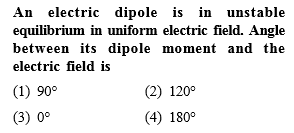You’ve reached the end of your free Videos limit.
#10 | Electric Field Superposition : 2 Electric Dipole
(Physics) > Electric Charges and Fields
Related Practice Questions :Complete Question Bank + Test Series
Complete Question Bank

Difficulty Level:

Eight dipoles of charges of magnitude e are placed inside a cube. The total electric flux coming out of the cube will be

(1) $\frac{8e}{{\epsilon }_{0}}$

(2) $\frac{16e}{{\epsilon }_{0}}$

(3) $\frac{e}{{\epsilon }_{0}}$

(4) Zero

Complete Question Bank + Test Series
Complete Question Bank

Difficulty Level:

An electric dipole is place at an angle of ${30}^{\circ }$ with an electric field intensity 2$×{10}^{5}$ N/C. it experiences a torque equal to 4 Nm. The charge on the diploe, if the dipole length is 2 cm, is

(a) 8 mC              (b) 2 mC

(c) 5 mC              (d) 7 $\mu$

Complete Question Bank + Test Series
Complete Question Bank

Difficulty Level:

An electric dipole of moment p is placed in an electric field of intensity E. The dipole acquires a position such that the axis of the dipole makes an angle $\theta$ with the direction of the field. Assuming that the potential energy of the dipole to be zero when $\theta =90°$,the torque and the potential energy of the dipole will respectively be

(a)

(b)

(c)

(d)